Successfully reported this slideshow.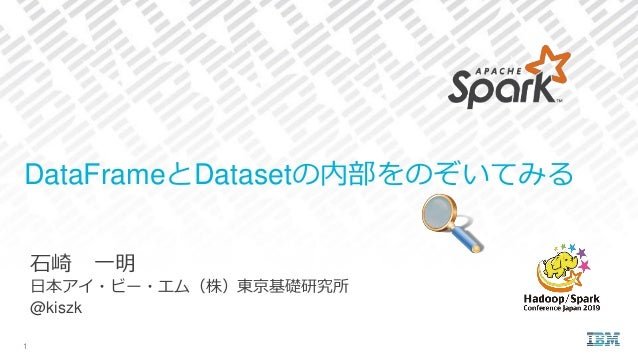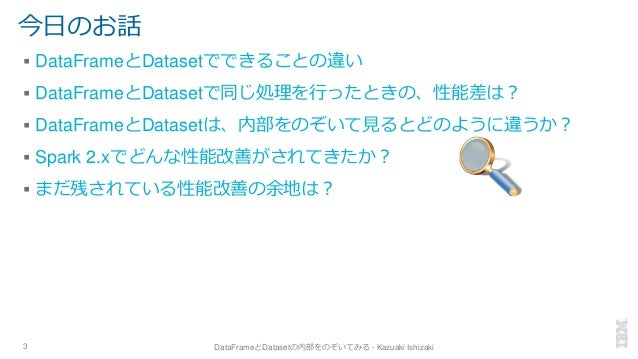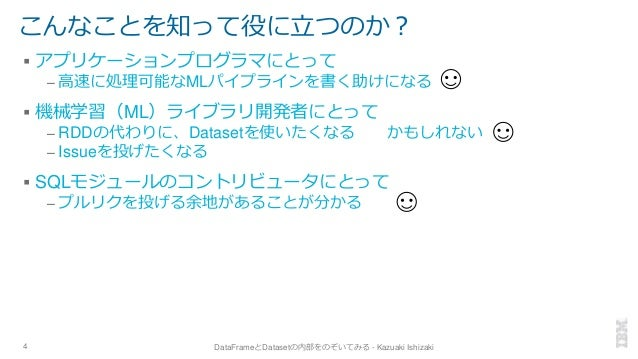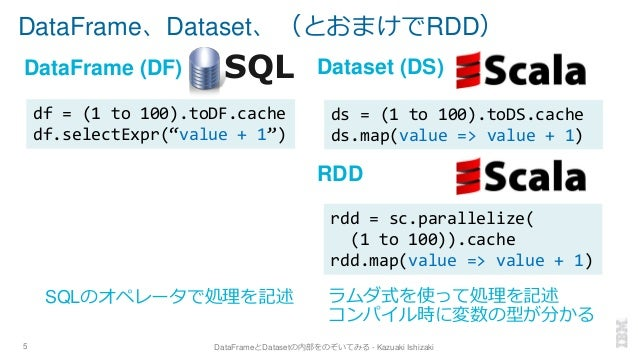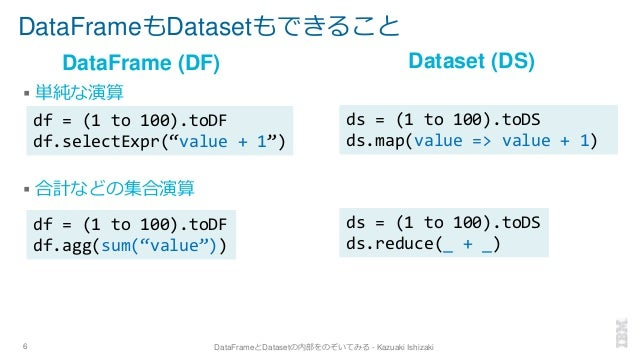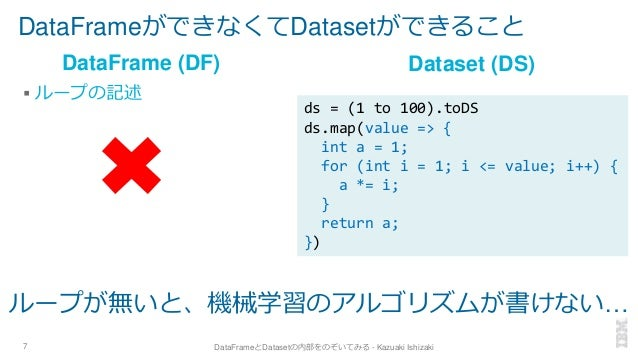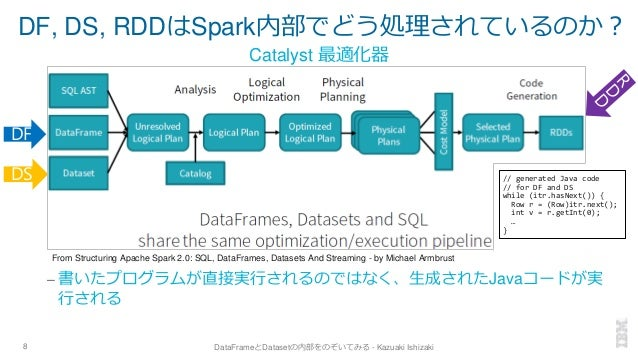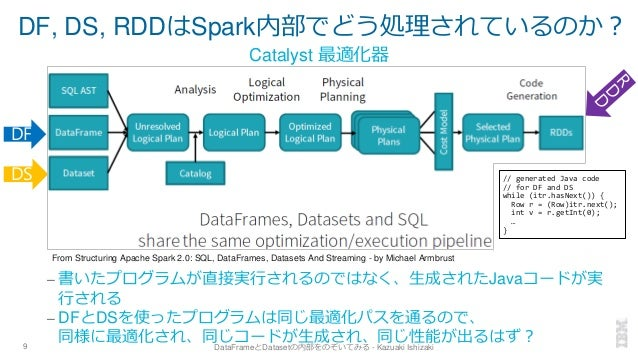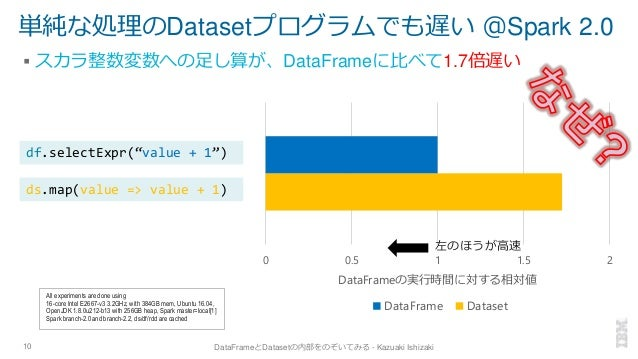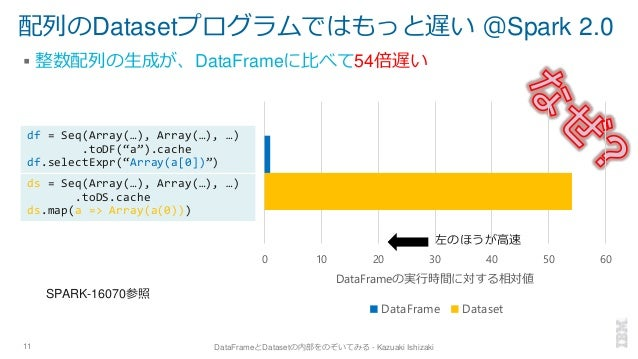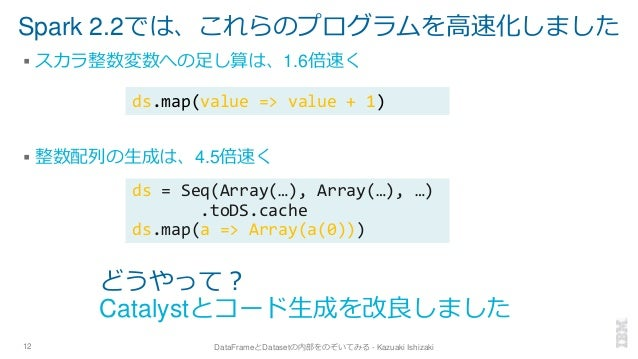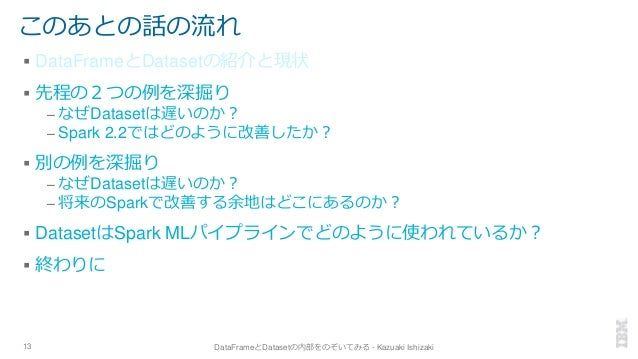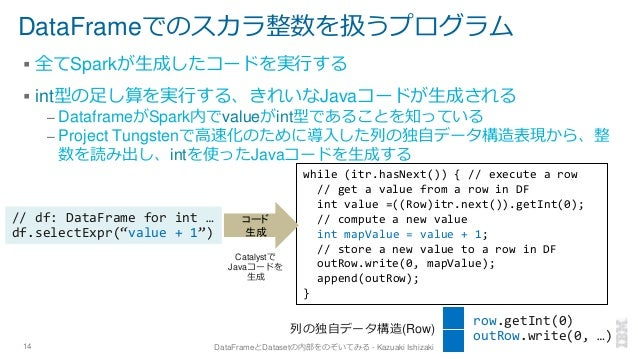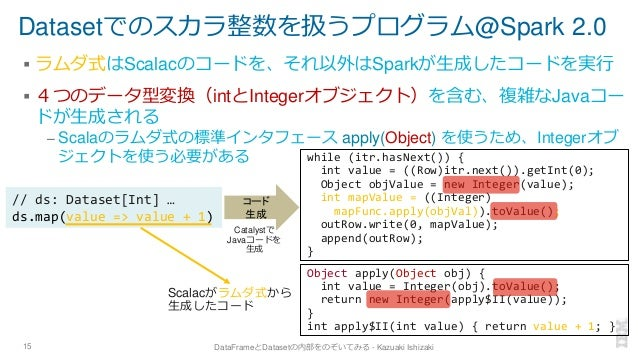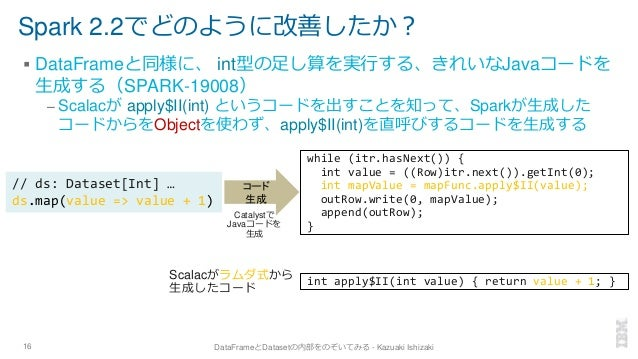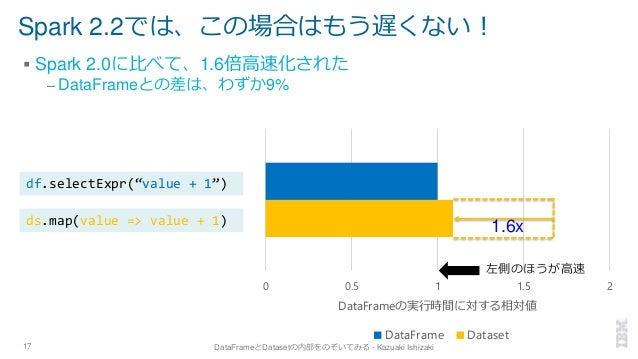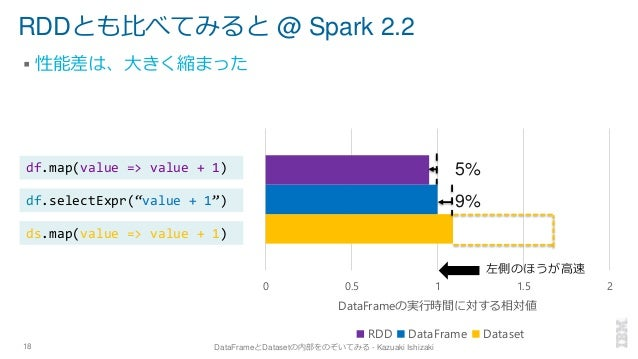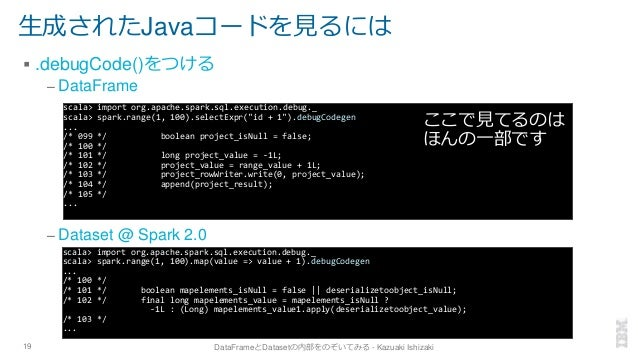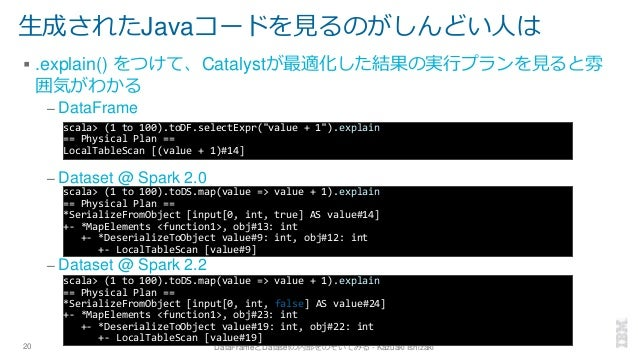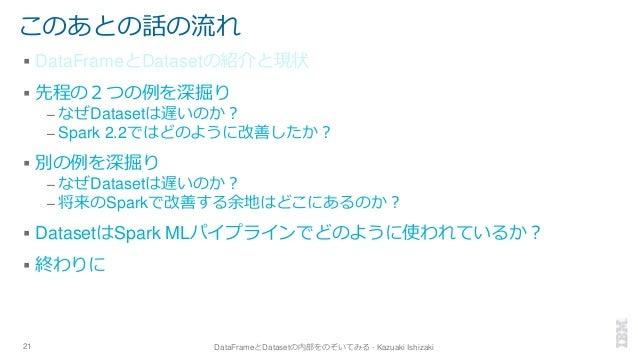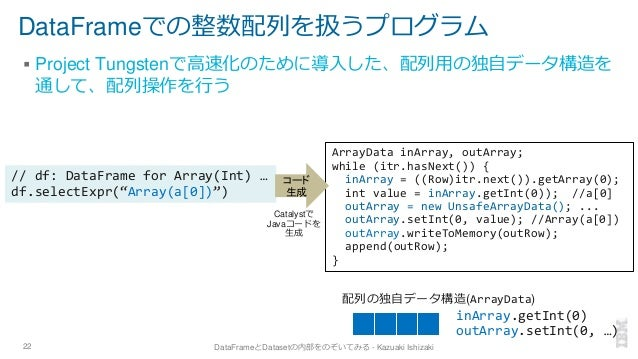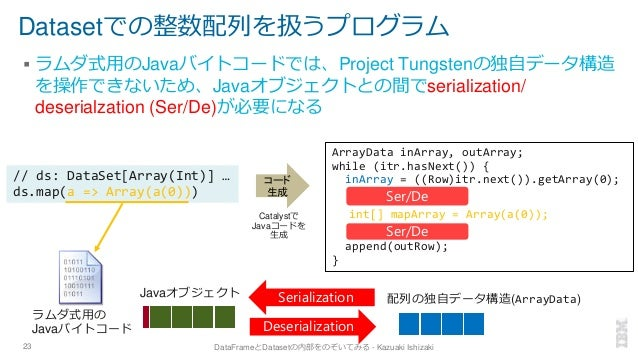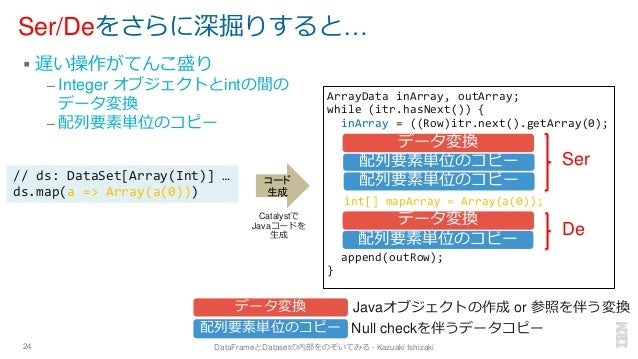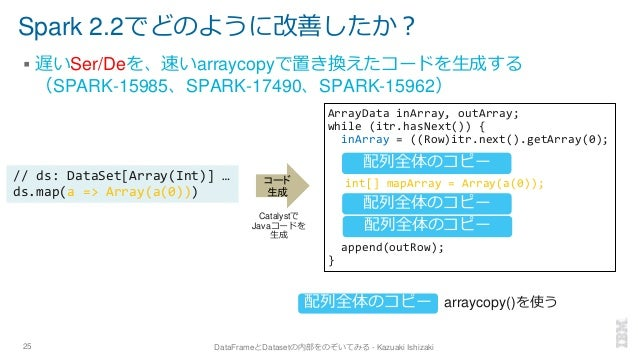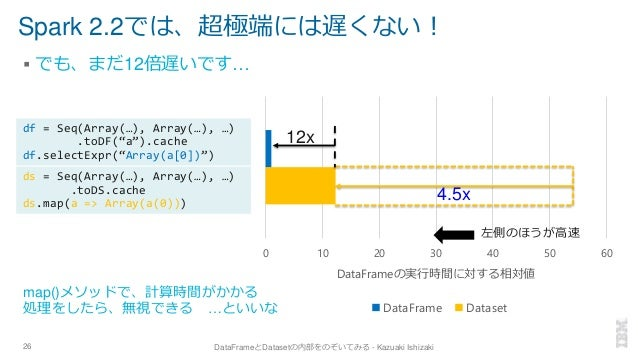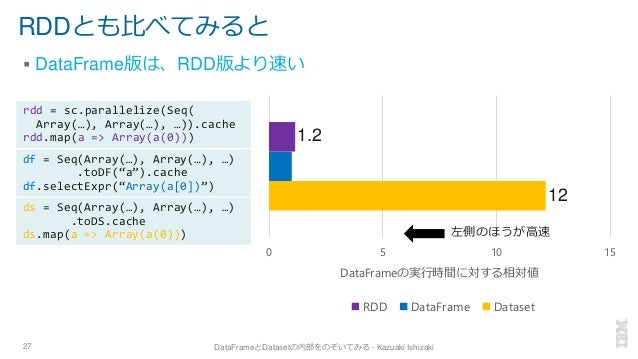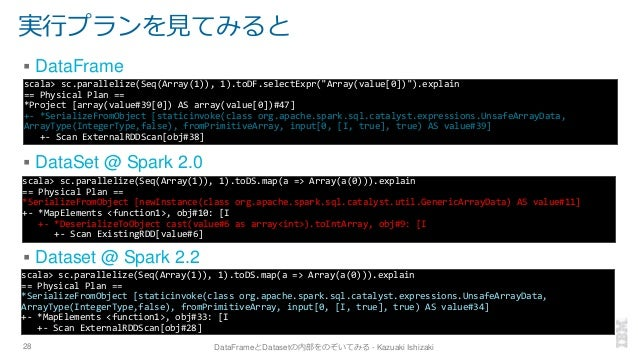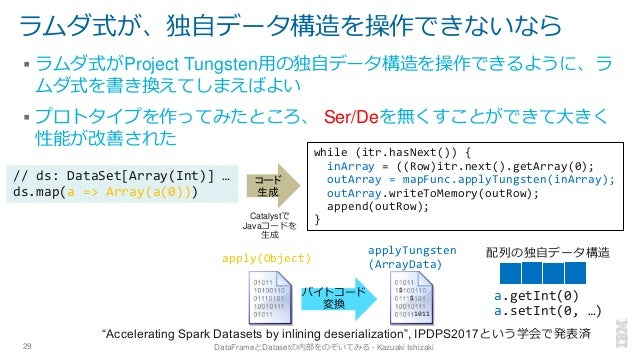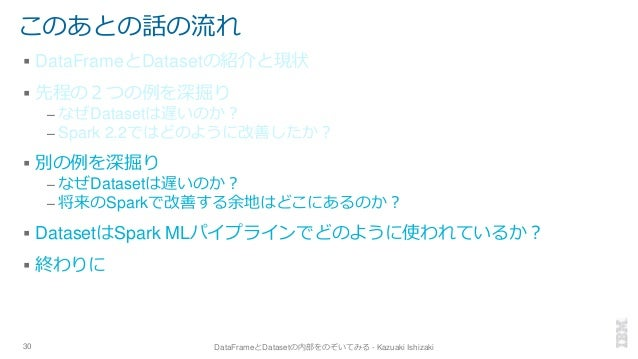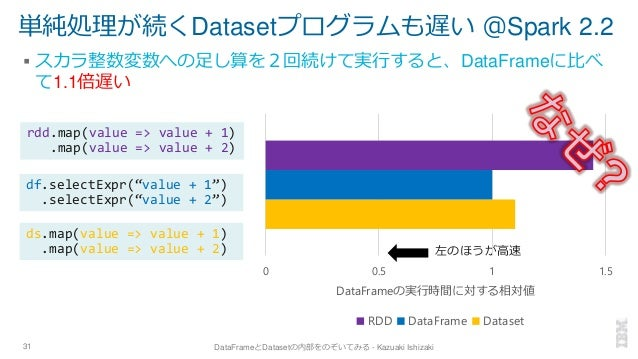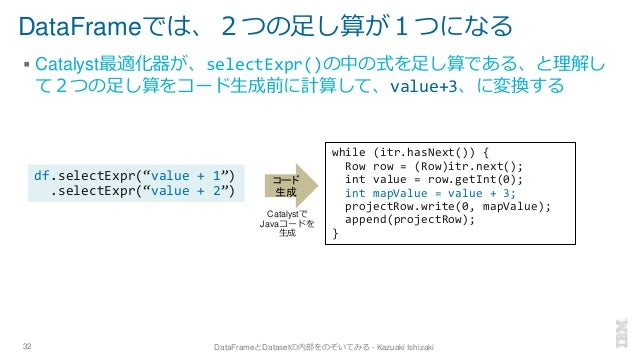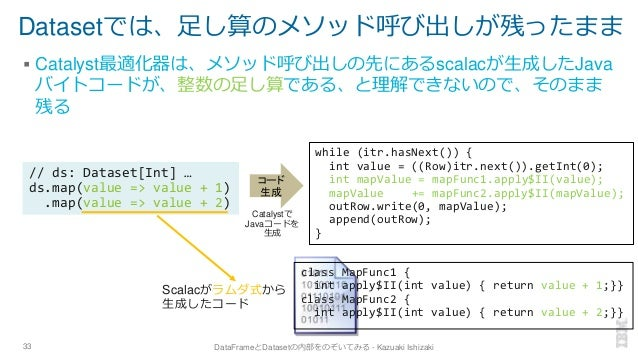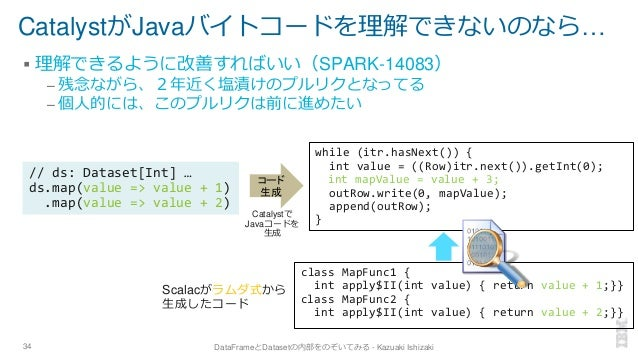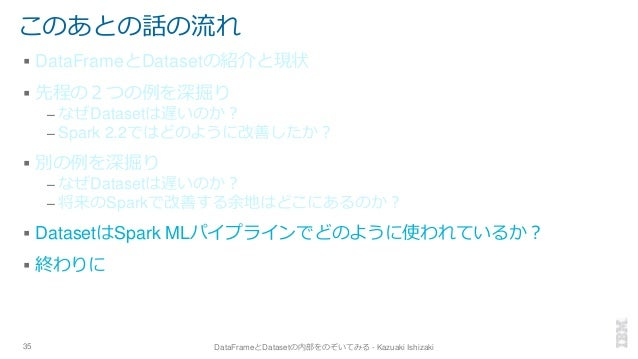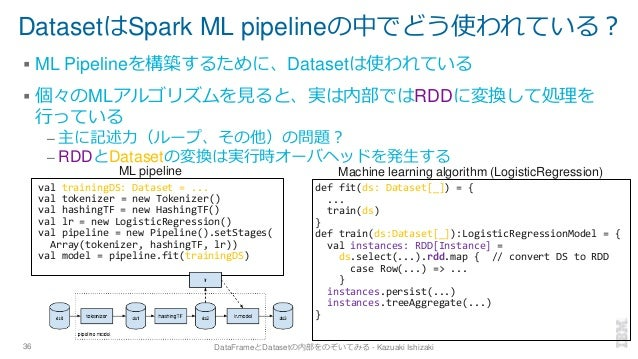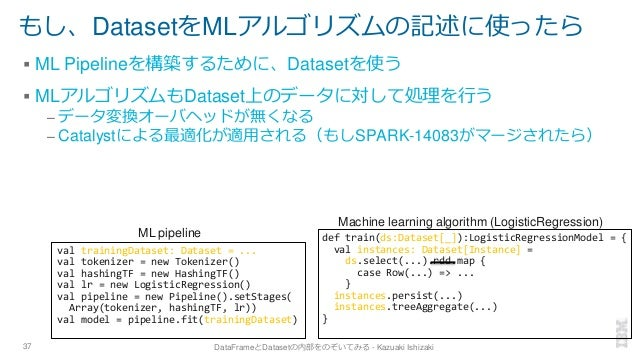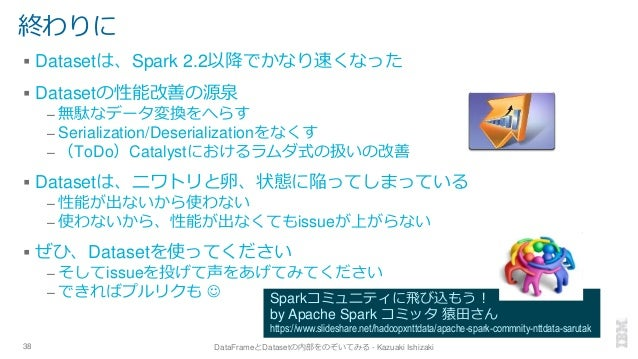Upcoming SlideShare
×

# hscj2019_ishizaki_public

「DataFrameとDatasetの内部をのぞいてみる」という内容の発表を、Hadoop / Spark Coference Japan 2019で行いました

• Full Name
Comment goes here.

Are you sure you want to Yes No• Be the first to comment

### hscj2019_ishizaki_public

1. 1. 石崎 一明 日本アイ・ビー・エム（株）東京基礎研究所 @kiszk DataFrameとDatasetの内部をのぞいてみる 1
2. 2. About Me – Kazuaki Ishizaki ▪ IBM Research – Tokyoで研究員をしています https://ibm.biz/ishizaki – コンパイラ最適化、言語処理系実装、並列処理、が専門 ▪ 1996年より、IBM Java（今はOpen J9）の実装にかかわる – 特に、PowerPC用の実行時コンパイラの実装リード ▪ 2018年9月より、Apache Sparkコミッタ（SQLモジュール） – 現在、Apache Sparkコミッタは日本に４人 ▪ 2018年よりACM Distinguished Member ▪ SNS – @kiszk – Slideshare: https://www.slideshare.net/ishizaki 2 DataFrameとDatasetの内部をのぞいてみる - Kazuaki Ishizaki
3. 3. 今日のお話 ▪ DataFrameとDatasetでできることの違い ▪ DataFrameとDatasetで同じ処理を行ったときの、性能差は？ ▪ DataFrameとDatasetは、内部をのぞいて見るとどのように違うか？ ▪ Spark 2.xでどんな性能改善がされてきたか？ ▪ まだ残されている性能改善の余地は？ 3 DataFrameとDatasetの内部をのぞいてみる - Kazuaki Ishizaki
4. 4. こんなことを知って役に立つのか？ ▪ アプリケーションプログラマにとって – 高速に処理可能なMLパイプラインを書く助けになる ▪ 機械学習（ML）ライブラリ開発者にとって – RDDの代わりに、Datasetを使いたくなる かもしれない – Issueを投げたくなる ▪ SQLモジュールのコントリビュータにとって – プルリクを投げる余地があることが分かる 4 DataFrameとDatasetの内部をのぞいてみる - Kazuaki Ishizaki
5. 5. DataFrame、Dataset、（とおまけでRDD） 5 DataFrameとDatasetの内部をのぞいてみる - Kazuaki Ishizaki SQLDataFrame (DF) Dataset (DS) RDD ラムダ式を使って処理を記述 コンパイル時に変数の型が分かる SQLのオペレータで処理を記述 df = (1 to 100).toDF.cache df.selectExpr(“value + 1”) ds = (1 to 100).toDS.cache ds.map(value => value + 1) rdd = sc.parallelize( (1 to 100)).cache rdd.map(value => value + 1)
6. 6. DataFrameもDatasetもできること ▪ 単純な演算 ▪ 合計などの集合演算 6 DataFrameとDatasetの内部をのぞいてみる - Kazuaki Ishizaki DataFrame (DF) Dataset (DS) df = (1 to 100).toDF df.agg(sum(“value”)) ds = (1 to 100).toDS ds.reduce(_ + _) ds = (1 to 100).toDS ds.map(value => value + 1) df = (1 to 100).toDF df.selectExpr(“value + 1”)
7. 7. DataFrameができなくてDatasetができること ▪ ループの記述 7 DataFrameとDatasetの内部をのぞいてみる - Kazuaki Ishizaki DataFrame (DF) Dataset (DS) ds = (1 to 100).toDS ds.map(value => { int a = 1; for (int i = 1; i <= value; i++) { a *= i; } return a; }) ループが無いと、機械学習のアルゴリズムが書けない…
8. 8. DF, DS, RDDはSpark内部でどう処理されているのか？ – 書いたプログラムが直接実行されるのではなく、生成されたJavaコードが実 行される 8 DataFrameとDatasetの内部をのぞいてみる - Kazuaki Ishizaki From Structuring Apache Spark 2.0: SQL, DataFrames, Datasets And Streaming - by Michael Armbrust DS DF Catalyst 最適化器 // generated Java code // for DF and DS while (itr.hasNext()) { Row r = (Row)itr.next(); int v = r.getInt(0); … }
9. 9. DF, DS, RDDはSpark内部でどう処理されているのか？ – 書いたプログラムが直接実行されるのではなく、生成されたJavaコードが実 行される – DFとDSを使ったプログラムは同じ最適化パスを通るので、 同様に最適化され、同じコードが生成され、同じ性能が出るはず？9 DataFrameとDatasetの内部をのぞいてみる - Kazuaki Ishizaki From Structuring Apache Spark 2.0: SQL, DataFrames, Datasets And Streaming - by Michael Armbrust DS DF Catalyst 最適化器 // generated Java code // for DF and DS while (itr.hasNext()) { Row r = (Row)itr.next(); int v = r.getInt(0); … }
10. 10. 単純な処理のDatasetプログラムでも遅い ＠Spark 2.0 ▪ スカラ整数変数への足し算が、DataFrameに比べて1.7倍遅い 10 DataFrameとDatasetの内部をのぞいてみる - Kazuaki Ishizaki 0 0.5 1 1.5 2 DataFrameの実行時間に対する相対値 DataFrame Dataset All experiments are done using 16-core Intel E2667-v3 3.2GHz, with 384GB mem, Ubuntu 16.04, OpenJDK 1.8.0u212-b13 with 256GB heap, Spark master=local Spark branch-2.0 and branch-2.2, ds/df/rdd are cached 左のほうが高速 ds.map(value => value + 1) df.selectExpr(“value + 1”)
11. 11. 配列のDatasetプログラムではもっと遅い ＠Spark 2.0 ▪ 整数配列の生成が、DataFrameに比べて54倍遅い 11 DataFrameとDatasetの内部をのぞいてみる - Kazuaki Ishizaki 左のほうが高速 0 10 20 30 40 50 60 DataFrameの実行時間に対する相対値 DataFrame Dataset SPARK-16070参照 ds = Seq(Array(…), Array(…), …) .toDS.cache ds.map(a => Array(a(0))) df = Seq(Array(…), Array(…), …) .toDF(“a”).cache df.selectExpr(“Array(a)”)
12. 12. Spark 2.2では、これらのプログラムを高速化しました ▪ スカラ整数変数への足し算は、1.6倍速く ▪ 整数配列の生成は、4.5倍速く 12 DataFrameとDatasetの内部をのぞいてみる - Kazuaki Ishizaki どうやって？ Catalystとコード生成を改良しました ds.map(value => value + 1) ds = Seq(Array(…), Array(…), …) .toDS.cache ds.map(a => Array(a(0)))
13. 13. このあとの話の流れ ▪ DataFrameとDatasetの紹介と現状 ▪ 先程の２つの例を深掘り – なぜDatasetは遅いのか？ – Spark 2.2ではどのように改善したか？ ▪ 別の例を深掘り – なぜDatasetは遅いのか？ – 将来のSparkで改善する余地はどこにあるのか？ ▪ DatasetはSpark MLパイプラインでどのように使われているか？ ▪ 終わりに 13 DataFrameとDatasetの内部をのぞいてみる - Kazuaki Ishizaki
14. 14. DataFrameでのスカラ整数を扱うプログラム ▪ 全てSparkが生成したコードを実行する ▪ int型の足し算を実行する、きれいなJavaコードが生成される – DataframeがSpark内でvalueがint型であることを知っている – Project Tungstenで高速化のために導入した列の独自データ構造表現から、整 数を読み出し、intを使ったJavaコードを生成する 14 while (itr.hasNext()) { // execute a row // get a value from a row in DF int value =((Row)itr.next()).getInt(0); // compute a new value int mapValue = value + 1; // store a new value to a row in DF outRow.write(0, mapValue); append(outRow); } // df: DataFrame for int … df.selectExpr(“value + 1”) コード 生成 DataFrameとDatasetの内部をのぞいてみる - Kazuaki Ishizaki Catalystで Javaコードを 生成 列の独自データ構造(Row) row.getInt(0) outRow.write(0, …)
15. 15. Datasetでのスカラ整数を扱うプログラム@Spark 2.0 ▪ ラムダ式はScalacのコードを、それ以外はSparkが生成したコードを実行 ▪ ４つのデータ型変換（intとIntegerオブジェクト）を含む、複雑なJavaコー ドが生成される – Scalaのラムダ式の標準インタフェース apply(Object) を使うため、Integerオブ ジェクトを使う必要がある 15 コード 生成 DataFrameとDatasetの内部をのぞいてみる - Kazuaki Ishizaki Catalystで Javaコードを 生成 // ds: Dataset[Int] … ds.map(value => value + 1) while (itr.hasNext()) { int value = ((Row)itr.next()).getInt(0); Object objValue = new Integer(value); int mapValue = ((Integer) mapFunc.apply(objVal)).toValue(); outRow.write(0, mapValue); append(outRow); } Object apply(Object obj) { int value = Integer(obj).toValue(); return new Integer(apply\$II(value)); } int apply\$II(int value) { return value + 1; } Scalacがラムダ式から 生成したコード
16. 16. Spark 2.2でどのように改善したか？ ▪ DataFrameと同様に、 int型の足し算を実行する、きれいなJavaコードを 生成する（SPARK-19008） – Scalacが apply\$II(int) というコードを出すことを知って、Sparkが生成した コードからをObjectを使わず、apply\$II(int)を直呼びするコードを生成する 16 コード 生成 DataFrameとDatasetの内部をのぞいてみる - Kazuaki Ishizaki Catalystで Javaコードを 生成 // ds: Dataset[Int] … ds.map(value => value + 1) while (itr.hasNext()) { int value = ((Row)itr.next()).getInt(0); int mapValue = mapFunc.apply\$II(value); outRow.write(0, mapValue); append(outRow); } int apply\$II(int value) { return value + 1; } Scalacがラムダ式から 生成したコード
17. 17. Spark 2.2では、この場合はもう遅くない！ ▪ Spark 2.0に比べて、1.6倍高速化された – DataFrameとの差は、わずか9% 17 DataFrameとDatasetの内部をのぞいてみる - Kazuaki Ishizaki 0 0.5 1 1.5 2 DataFrameの実行時間に対する相対値 DataFrame Dataset 左側のほうが高速 1.6xds.map(value => value + 1) df.selectExpr(“value + 1”)
18. 18. RDDとも比べてみると @ Spark 2.2 ▪ 性能差は、大きく縮まった 18 DataFrameとDatasetの内部をのぞいてみる - Kazuaki Ishizaki 0 0.5 1 1.5 2 DataFrameの実行時間に対する相対値 RDD DataFrame Dataset 9% 5% 左側のほうが高速 ds.map(value => value + 1) df.selectExpr(“value + 1”) df.map(value => value + 1)
19. 19. 生成されたJavaコードを見るには ▪ .debugCode()をつける – DataFrame – Dataset @ Spark 2.0 19 DataFrameとDatasetの内部をのぞいてみる - Kazuaki Ishizaki scala> import org.apache.spark.sql.execution.debug._ scala> spark.range(1, 100).selectExpr("id + 1").debugCodegen ... /* 099 */ boolean project_isNull = false; /* 100 */ /* 101 */ long project_value = -1L; /* 102 */ project_value = range_value + 1L; /* 103 */ project_rowWriter.write(0, project_value); /* 104 */ append(project_result); /* 105 */ ... scala> import org.apache.spark.sql.execution.debug._ scala> spark.range(1, 100).map(value => value + 1).debugCodegen ... /* 100 */ /* 101 */ boolean mapelements_isNull = false || deserializetoobject_isNull; /* 102 */ final long mapelements_value = mapelements_isNull ? -1L : (Long) mapelements_value1.apply(deserializetoobject_value); /* 103 */ ... ここで見てるのは ほんの一部です
20. 20. 生成されたJavaコードを見るのがしんどい人は ▪ .explain() をつけて、Catalystが最適化した結果の実行プランを見ると雰 囲気がわかる – DataFrame – Dataset @ Spark 2.0 – Dataset @ Spark 2.2 20 DataFrameとDatasetの内部をのぞいてみる - Kazuaki Ishizaki scala> (1 to 100).toDF.selectExpr("value + 1").explain == Physical Plan == LocalTableScan [(value + 1)#14] scala> (1 to 100).toDS.map(value => value + 1).explain == Physical Plan == *SerializeFromObject [input[0, int, false] AS value#24] +- *MapElements <function1>, obj#23: int +- *DeserializeToObject value#19: int, obj#22: int +- LocalTableScan [value#19] scala> (1 to 100).toDS.map(value => value + 1).explain == Physical Plan == *SerializeFromObject [input[0, int, true] AS value#14] +- *MapElements <function1>, obj#13: int +- *DeserializeToObject value#9: int, obj#12: int +- LocalTableScan [value#9]
21. 21. このあとの話の流れ ▪ DataFrameとDatasetの紹介と現状 ▪ 先程の２つの例を深掘り – なぜDatasetは遅いのか？ – Spark 2.2ではどのように改善したか？ ▪ 別の例を深掘り – なぜDatasetは遅いのか？ – 将来のSparkで改善する余地はどこにあるのか？ ▪ DatasetはSpark MLパイプラインでどのように使われているか？ ▪ 終わりに 21 DataFrameとDatasetの内部をのぞいてみる - Kazuaki Ishizaki
22. 22. DataFrameでの整数配列を扱うプログラム ▪ Project Tungstenで高速化のために導入した、配列用の独自データ構造を 通して、配列操作を行う 22 DataFrameとDatasetの内部をのぞいてみる - Kazuaki Ishizaki ArrayData inArray, outArray; while (itr.hasNext()) { inArray = ((Row)itr.next()).getArray(0); int value = inArray.getInt(0)); //a outArray = new UnsafeArrayData(); ... outArray.setInt(0, value); //Array(a) outArray.writeToMemory(outRow); append(outRow); } 配列の独自データ構造(ArrayData) inArray.getInt(0) outArray.setInt(0, …) // df: DataFrame for Array(Int) … df.selectExpr(“Array(a)”) Catalystで Javaコードを 生成 コード 生成
23. 23. Datasetでの整数配列を扱うプログラム ▪ ラムダ式用のJavaバイトコードでは、Project Tungstenの独自データ構造 を操作できないため、Javaオブジェクトとの間でserialization/ deserialzation (Ser/De)が必要になる 23 DataFrameとDatasetの内部をのぞいてみる - Kazuaki Ishizaki ArrayData inArray, outArray; while (itr.hasNext()) { inArray = ((Row)itr.next()).getArray(0); append(outRow); } 配列の独自データ構造(ArrayData) // ds: DataSet[Array(Int)] … ds.map(a => Array(a(0))) Catalystで Javaコードを 生成 コード 生成 Ser/De int[] mapArray = Array(a(0)); Ser/De Javaオブジェクト ラムダ式用の Javaバイトコード Deserialization Serialization
24. 24. Ser/Deをさらに深掘りすると… ▪ 遅い操作がてんこ盛り – Integer オブジェクトとintの間の データ変換 – 配列要素単位のコピー 24 DataFrameとDatasetの内部をのぞいてみる - Kazuaki Ishizaki // ds: DataSet[Array(Int)] … ds.map(a => Array(a(0))) Catalystで Javaコードを 生成 コード 生成 ArrayData inArray, outArray; while (itr.hasNext()) { inArray = ((Row)itr.next().getArray(0); append(outRow); } データ変換 配列要素単位のコピー 配列要素単位のコピー データ変換 配列要素単位のコピー Null checkを伴うデータコピー int[] mapArray = Array(a(0)); データ変換 配列要素単位のコピー Ser De Javaオブジェクトの作成 or 参照を伴う変換
25. 25. Spark 2.2でどのように改善したか？ ▪ 遅いSer/Deを、速いarraycopyで置き換えたコードを生成する （SPARK-15985、SPARK-17490、SPARK-15962） 25 DataFrameとDatasetの内部をのぞいてみる - Kazuaki Ishizaki // ds: DataSet[Array(Int)] … ds.map(a => Array(a(0))) Catalystで Javaコードを 生成 コード 生成 ArrayData inArray, outArray; while (itr.hasNext()) { inArray = ((Row)itr.next().getArray(0); append(outRow); } int[] mapArray = Array(a(0)); arraycopy()を使う配列全体のコピー 配列全体のコピー 配列全体のコピー 配列全体のコピー
26. 26. Spark 2.2では、超極端には遅くない！ ▪ でも、まだ12倍遅いです… 26 DataFrameとDatasetの内部をのぞいてみる - Kazuaki Ishizaki 0 10 20 30 40 50 60 DataFrameの実行時間に対する相対値 DataFrame Dataset 4.5x 12x 左側のほうが高速 ds = Seq(Array(…), Array(…), …) .toDS.cache ds.map(a => Array(a(0))) df = Seq(Array(…), Array(…), …) .toDF(“a”).cache df.selectExpr(“Array(a)”) map()メソッドで、計算時間がかかる 処理をしたら、無視できる …といいな
27. 27. RDDとも比べてみると ▪ DataFrame版は、RDD版より速い 27 DataFrameとDatasetの内部をのぞいてみる - Kazuaki Ishizaki ds = Seq(Array(…), Array(…), …) .toDS.cache ds.map(a => Array(a(0))) df = Seq(Array(…), Array(…), …) .toDF(“a”).cache df.selectExpr(“Array(a)”) 0 5 10 15 DataFrameの実行時間に対する相対値 RDD DataFrame Dataset 1.2 12 左側のほうが高速 rdd = sc.parallelize(Seq( Array(…), Array(…), …)).cache rdd.map(a => Array(a(0)))
28. 28. 実行プランを見てみると ▪ DataFrame ▪ DataSet @ Spark 2.0 ▪ Dataset @ Spark 2.2 28 DataFrameとDatasetの内部をのぞいてみる - Kazuaki Ishizaki scala> sc.parallelize(Seq(Array(1)), 1).toDF.selectExpr("Array(value)").explain == Physical Plan == *Project [array(value#39) AS array(value)#47] +- *SerializeFromObject [staticinvoke(class org.apache.spark.sql.catalyst.expressions.UnsafeArrayData, ArrayType(IntegerType,false), fromPrimitiveArray, input[0, [I, true], true) AS value#39] +- Scan ExternalRDDScan[obj#38] scala> sc.parallelize(Seq(Array(1)), 1).toDS.map(a => Array(a(0))).explain == Physical Plan == *SerializeFromObject [newInstance(class org.apache.spark.sql.catalyst.util.GenericArrayData) AS value#11] +- *MapElements <function1>, obj#10: [I +- *DeserializeToObject cast(value#6 as array<int>).toIntArray, obj#9: [I +- Scan ExistingRDD[value#6] scala> sc.parallelize(Seq(Array(1)), 1).toDS.map(a => Array(a(0))).explain == Physical Plan == *SerializeFromObject [staticinvoke(class org.apache.spark.sql.catalyst.expressions.UnsafeArrayData, ArrayType(IntegerType,false), fromPrimitiveArray, input[0, [I, true], true) AS value#34] +- *MapElements <function1>, obj#33: [I +- Scan ExternalRDDScan[obj#28]
29. 29. ラムダ式が、独自データ構造を操作できないなら ▪ ラムダ式がProject Tungsten用の独自データ構造を操作できるように、ラ ムダ式を書き換えてしまえばよい ▪ プロトタイプを作ってみたところ、 Ser/Deを無くすことができて大きく 性能が改善された 29 DataFrameとDatasetの内部をのぞいてみる - Kazuaki Ishizaki while (itr.hasNext()) { inArray = ((Row)itr.next().getArray(0); outArray = mapFunc.applyTungsten(inArray); outArray.writeToMemory(outRow); append(outRow); } // ds: DataSet[Array(Int)] … ds.map(a => Array(a(0))) Catalystで Javaコードを 生成 コード 生成 “Accelerating Spark Datasets by inlining deserialization”, IPDPS2017という学会で発表済 配列の独自データ構造 1 1 apply(Object) 1011 applyTungsten (ArrayData) バイトコード 変換 a.getInt(0) a.setInt(0, …)
30. 30. このあとの話の流れ ▪ DataFrameとDatasetの紹介と現状 ▪ 先程の２つの例を深掘り – なぜDatasetは遅いのか？ – Spark 2.2ではどのように改善したか？ ▪ 別の例を深掘り – なぜDatasetは遅いのか？ – 将来のSparkで改善する余地はどこにあるのか？ ▪ DatasetはSpark MLパイプラインでどのように使われているか？ ▪ 終わりに 30 DataFrameとDatasetの内部をのぞいてみる - Kazuaki Ishizaki
31. 31. 単純処理が続くDatasetプログラムも遅い ＠Spark 2.2 ▪ スカラ整数変数への足し算を２回続けて実行すると、DataFrameに比べ て1.1倍遅い 31 DataFrameとDatasetの内部をのぞいてみる - Kazuaki Ishizaki 左のほうが高速 ds.map(value => value + 1) .map(value => value + 2) df.selectExpr(“value + 1”) .selectExpr(“value + 2”) rdd.map(value => value + 1) .map(value => value + 2) 0 0.5 1 1.5 DataFrameの実行時間に対する相対値 RDD DataFrame Dataset
32. 32. DataFrameでは、２つの足し算が１つになる ▪ Catalyst最適化器が、selectExpr()の中の式を足し算である、と理解し て２つの足し算をコード生成前に計算して、value+3、に変換する 32 DataFrameとDatasetの内部をのぞいてみる - Kazuaki Ishizaki while (itr.hasNext()) { Row row = (Row)itr.next(); int value = row.getInt(0); int mapValue = value + 3; projectRow.write(0, mapValue); append(projectRow); } df.selectExpr(“value + 1”) .selectExpr(“value + 2”) Catalystで Javaコードを 生成 コード 生成
33. 33. Datasetでは、足し算のメソッド呼び出しが残ったまま ▪ Catalyst最適化器は、メソッド呼び出しの先にあるscalacが生成したJava バイトコードが、整数の足し算である、と理解できないので、そのまま 残る 33 DataFrameとDatasetの内部をのぞいてみる - Kazuaki Ishizaki while (itr.hasNext()) { int value = ((Row)itr.next()).getInt(0); int mapValue = mapFunc1.apply\$II(value); mapValue += mapFunc2.apply\$II(mapValue); outRow.write(0, mapValue); append(outRow); } // ds: Dataset[Int] … ds.map(value => value + 1) .map(value => value + 2) Catalystで Javaコードを 生成 コード 生成 class MapFunc1 { int apply\$II(int value) { return value + 1;}} class MapFunc2 { int apply\$II(int value) { return value + 2;}} Scalacがラムダ式から 生成したコード
34. 34. CatalystがJavaバイトコードを理解できないのなら… ▪ 理解できるように改善すればいい（SPARK-14083） – 残念ながら、２年近く塩漬けのプルリクとなってる – 個人的には、このプルリクは前に進めたい 34 DataFrameとDatasetの内部をのぞいてみる - Kazuaki Ishizaki while (itr.hasNext()) { int value = ((Row)itr.next()).getInt(0); int mapValue = value + 3; outRow.write(0, mapValue); append(outRow); } // ds: Dataset[Int] … ds.map(value => value + 1) .map(value => value + 2) Catalystで Javaコードを 生成 コード 生成 class MapFunc1 { int apply\$II(int value) { return value + 1;}} class MapFunc2 { int apply\$II(int value) { return value + 2;}} Scalacがラムダ式から 生成したコード
35. 35. このあとの話の流れ ▪ DataFrameとDatasetの紹介と現状 ▪ 先程の２つの例を深掘り – なぜDatasetは遅いのか？ – Spark 2.2ではどのように改善したか？ ▪ 別の例を深掘り – なぜDatasetは遅いのか？ – 将来のSparkで改善する余地はどこにあるのか？ ▪ DatasetはSpark MLパイプラインでどのように使われているか？ ▪ 終わりに 35 DataFrameとDatasetの内部をのぞいてみる - Kazuaki Ishizaki
36. 36. DatasetはSpark ML pipelineの中でどう使われている？ ▪ ML Pipelineを構築するために、Datasetは使われている ▪ 個々のMLアルゴリズムを見ると、実は内部ではRDDに変換して処理を 行っている – 主に記述力（ループ、その他）の問題？ – RDDとDatasetの変換は実行時オーバヘッドを発生する 36 DataFrameとDatasetの内部をのぞいてみる - Kazuaki Ishizaki val trainingDS: Dataset = ... val tokenizer = new Tokenizer() val hashingTF = new HashingTF() val lr = new LogisticRegression() val pipeline = new Pipeline().setStages( Array(tokenizer, hashingTF, lr)) val model = pipeline.fit(trainingDS) def fit(ds: Dataset[_]) = { ... train(ds) } def train(ds:Dataset[_]):LogisticRegressionModel = { val instances: RDD[Instance] = ds.select(...).rdd.map { // convert DS to RDD case Row(...) => ... } instances.persist(...) instances.treeAggregate(...) } ML pipeline Machine learning algorithm (LogisticRegression)
37. 37. もし、DatasetをMLアルゴリズムの記述に使ったら ▪ ML Pipelineを構築するために、Datasetを使う ▪ MLアルゴリズムもDataset上のデータに対して処理を行う – データ変換オーバヘッドが無くなる – Catalystによる最適化が適用される（もしSPARK-14083がマージされたら） 37 DataFrameとDatasetの内部をのぞいてみる - Kazuaki Ishizaki val trainingDataset: Dataset = ... val tokenizer = new Tokenizer() val hashingTF = new HashingTF() val lr = new LogisticRegression() val pipeline = new Pipeline().setStages( Array(tokenizer, hashingTF, lr)) val model = pipeline.fit(trainingDataset) def train(ds:Dataset[_]):LogisticRegressionModel = { val instances: Dataset[Instance] = ds.select(...).rdd.map { case Row(...) => ... } instances.persist(...) instances.treeAggregate(...) } ML pipeline Machine learning algorithm (LogisticRegression)
38. 38. 終わりに ▪ Datasetは、Spark 2.2以降でかなり速くなった ▪ Datasetの性能改善の源泉 – 無駄なデータ変換をへらす – Serialization/Deserializationをなくす – （ToDo）Catalystにおけるラムダ式の扱いの改善 ▪ Datasetは、ニワトリと卵、状態に陥ってしまっている – 性能が出ないから使わない – 使わないから、性能が出なくてもissueが上がらない ▪ ぜひ、Datasetを使ってください – そしてissueを投げて声をあげてみてください – できればプルリクも ☺ 38 DataFrameとDatasetの内部をのぞいてみる - Kazuaki Ishizaki Sparkコミュニティに飛び込もう！ by Apache Spark コミッタ 猿田さん https://www.slideshare.net/hadoopxnttdata/apache-spark-commnity-nttdata-sarutak
39. 39. Thank you ▪ @sameeragarwal, @hvanhovell, @gatorsmile, @cloud-fan, @ueshin, @davies, @rxin, @joshrosen, @maropu, @viirya ▪ IBM CODAIT 39 DataFrameとDatasetの内部をのぞいてみる - Kazuaki Ishizaki

### Be the first to comment

•#### ShimpeiTakemoto

Mar. 14, 2019
•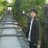#### otsumoo

Mar. 20, 2020

Total views

3,670

On Slideshare

0

From embeds

0

Number of embeds

1,615

17

Shares

0